mscroggs.co.uk
mscroggs.co.uksubscribe

# Blog

2020-03-31
Recently, you've probably seen a lot of graphs that look like this:
The graph above shows something that is growing exponentially: its equation is $$y=kr^x$$, for some constants $$k$$ and $$r$$. The value of the constant $$r$$ is very important, as it tells you how quickly the value is going to grow. Using a graph of some data, it is difficult to get an anywhere-near-accurate approximation of $$r$$.
The following plot shows three different exponentials. It's very difficult to say anything about them except that they grow very quickly above around $$x=15$$.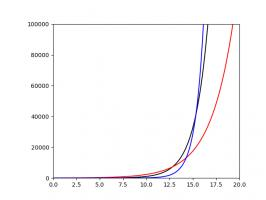$$y=2^x$$, $$y=40\times 1.5^x$$, and $$y=0.002\times3^x$$
It would be nice if we could plot these in a way that their important properties—such as the value of the ratio $$r$$—were more clearly evident from the graph. To do this, we start by taking the log of both sides of the equation:
$$\log y=\log(kr^x)$$
Using the laws of logs, this simplifies to:
$$\log y=\log k+x\log r$$
This is now the equation of a straight line, $$\hat{y}=m\hat{x}+c$$, with $$\hat{y}=\log y$$, $$\hat{x}=x$$, $$m=\log r$$ and $$c=\log k$$. So if we plot $$x$$ against $$\log y$$, we should get a straight line with gradient $$\log r$$. If we plot the same three exponentials as above using a log-scaled $$y$$-axis, we get: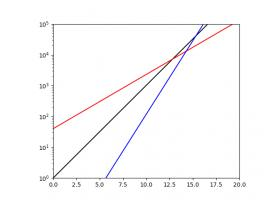$$y=2^x$$, $$y=40\times 1.5^x$$, and $$y=0.002\times3^x$$ with a log-scaled $$y$$-axis
From this picture alone, it is very clear that the blue exponential has the largest value of $$r$$, and we could quickly work out a decent approximation of this value by calculating 10 (or the base of the log used if using a different log) to the power of the gradient.

### Log-log plots

Exponential growth isn't the only situation where scaling the axes is beneficial. In my research in finite and boundary element methods, it is common that the error of the solution $$e$$ is given in terms of a grid parameter $$h$$ by a polynomial of the form $$e=ah^k$$, for some constants $$a$$ and $$k$$.
We are often interested in the value of the power $$k$$. If we plot $$e$$ against $$h$$, it's once again difficult to judge the value of $$k$$ from the graph alone. The following graph shows three polynomials.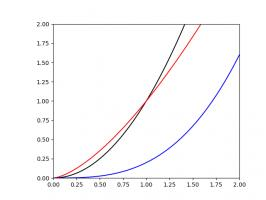$$y=x^2$$, $$y=x^{1.5}$$, and $$y=0.5x^3$$
Once again is is difficult to judge any of the important properties of these. To improve this, we once again begin by taking the log of each side of the equation:
$$\log e=\log (ah^k)$$
Applying the laws of logs this time gives:
$$\log e=\log a+k\log h$$
This is now the equation of a straight line, $$\hat{y}=m\hat{x}+c$$, with $$\hat{y}=\log e$$, $$\hat{x}=\log h$$, $$m=k$$ and $$c=\log a$$. So if we plot $$\log x$$ against $$\log y$$, we should get a straight line with gradient $$k$$.
Doing this for the same three curves as above gives the following plot.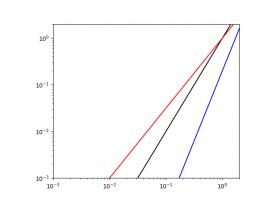$$y=x^2$$, $$y=x^{1.5}$$, and $$y=0.5x^3$$ with log-scaled $$x$$- and $$y$$-axes
It is easy to see that the blue line has the highest value of $$k$$ (as it has the highest gradient, and we could get a decent approximation of this value by finding the line's gradient.

As well as making it easier to get good approximations of important parameters, making curves into straight lines in this way also makes it easier to plot the trend of real data. Drawing accurate exponentials and polynomials is hard at the best of times; and real data will not exactly follow the curve, so drawing an exponential or quadratic of best fit will be an even harder task. By scaling the axes first though, this task simplifies to drawing a straight line through the data; this is much easier.
So next time you're struggling with an awkward curve, why not try turning it into a straight line first.

### Similar postsVisualising MENACE's learning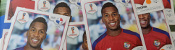World Cup stickers 2018, pt. 2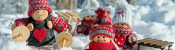Christmas (2020) is over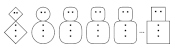Christmas card 2020

Comments in green were written by me. Comments in blue were not written by me.

Allowed HTML tags: <br> <a> <small> <b> <i> <s> <sup> <sub> <u> <spoiler> <ul> <ol> <li>
To prove you are not a spam bot, please type "segment" in the box below (case sensitive):
2019-12-27
In tonight's Royal Institution Christmas lecture, Hannah Fry and Matt Parker demonstrated how machine learning works using MENACE.
The copy of MENACE that appeared in the lecture was build and trained by me. During the training, I logged all the moved made by MENACE and the humans playing against them, and using this data I have created some visualisations of the machine's learning.
First up, here's a visualisation of the likelihood of MENACE choosing different moves as they play games. The thickness of each arrow represented the number of beads in the box corresponding to that move, so thicker arrows represent more likely moves.
The likelihood that MENACE will play each move.
There's an awful lot of arrows in this diagram, so it's clearer if we just visualise a few boxes. This animation shows how the number of beads in the first box changes over time.
You can see that MENACE learnt that they should always play in the centre first, an ends up with a large number of green beads and almost none of the other colours. The following animations show the number of beads changing in some other boxes.
MENACE learns that the top left is a good move.
MENACE learns that the middle right is a good move.
MENACE is very likely to draw from this position so learns that almost all the possible moves are good moves.
The numbers in these change less often, as they are not used in every game: they are only used when the game reached the positions shown on the boxes.
We can visualise MENACE's learning progress by plotting how the number of beads in the first box changes over time.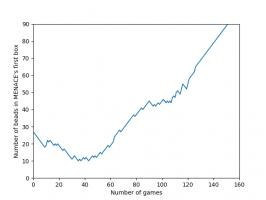The number of beads in MENACE's first box.
Alternatively, we could plot how the number of wins, loses and draws changes over time or view this as an animated bar chart.The number of games MENACE wins, loses and draws.
The number of games MENACE has won, lost and drawn.
If you have any ideas for other interesting ways to present this data, let me know in the comments below.

### Similar posts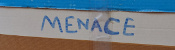Building MENACEs for other games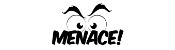MENACE at Manchester Science Festival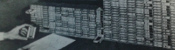MENACEMENACE in fiction

Comments in green were written by me. Comments in blue were not written by me.
@(anonymous): Have you been refreshing the page? Every time you refresh it resets MENACE to before it has learnt anything.

It takes around 80 games for MENACE to learn against the perfect AI. So it could be you've not left it playing for long enough? (Try turning the speed up to watch MENACE get better.)
Matthew
I have played around menace a bit and frankly it doesnt seem to be learning i occasionally play with it and it draws but againt the perfect ai you dont see as many draws, the perfect ai wins alot more
(anonymous)
@Colin: You can set MENACE playing against MENACE2 (MENACE that plays second) on the interactive MENACE. MENACE2's starting numbers of beads and incentives may need some tweaking to give it a chance though; I've been meaning to look into this in more detail at some point...
Matthew
Idle pondering (and something you may have covered elsewhere): what's the evolution as MENACE plays against itself? (Assuming MENACE can play both sides.)
Colin